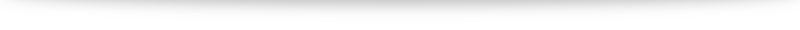# Q9b discussion

### The integration part

We have $\displaystyle \int \frac{1}{(1-x^2)(1+x^2)} \; \mathrm{d}x = t + C$. Since the denominator is so complicated, partial fractions is the way to go.
$$\frac{1}{(1-x)(1+x)(1+x^2)} = \frac{A}{1-x} + \frac{B}{1+x} + \frac{Cx+D}{1+x^2}.$$
Give it a go!

### The show part

Rate of increase at a constant rate $A=1$: So we have rate of increase = 1.

Rate of increase at a decrease is proportional to fourth power of $x$ with $k=1$: So we have rate of decrease $= x^4$.

Combining, we have $\displaystyle \frac{\mathrm{d}x}{\mathrm{d}t} = 1-x^4$. Factorizing it gives us the required result.